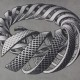# Lesson 23 Solution

Exercise 1)  Let$G$ be a group and let$a$ be any element in$G$.  Prove that the inverse of$a^{-1}$ is$a$.  I.e., show that “the inverse of the inverse is what you started with”.  (Hint: this is completely trivial from the definition of a group.  I.e., if we know that$a^{-1}$ is the inverse of$a$, then we simply take the properties that it has with respect to$a$ and note that they imply that$a$ also immediately has those properties with respect to$a^{-1}$.  This is mainly an exercise in getting the flow of logic correct and precise.

Solution:  As mentioned in the hint, this is mainly an exercise in getting the logic correct with arguments like these.  Thus, even though some steps may seem trivial, they’re indeed important to understand fully.  Let’s begin.

Pick any element$a$ in the group$G$, and let the element$a^{-1}$ denote a’s inverse (we know such an inverse exists because$G$ is a group, and inverses exist for all elements in a group by definition, and we know it’s unique by our work in this lesson).  Now we want to find the inverse of$a^{-1}$ since, after all,$a^{-1}$ is an element of$G$ and therefore itself has an inverse.  Well, we know that the inverse of$a^{-1}$ must be an element$b$ such that$a^{-1}\cdot b=b\cdot a^{-1}=e$, where$e\in G$ is the identity element (we’ve often denoted this by “0” as well).  But, we know that$a$ has the very same properties that$b$ does.  Therefore$b=a$, since we know that inverses are unique, and therefore$a$ is the inverse of$a^{-1}$, just as$a^{-1}$ is the inverse of$a$.

This shouldn’t be surprising since, for example, the inverse of$4$ is$-4$, and the inverse of$-4$ is$4$ (when the group is the integers with addition).  You should convince yourself that the groups we’ve discussed, and any that you may ever come up with, all have this property (as they must!).

Back to the lesson

On to lesson 24

### 2 Responses to Lesson 23 Solution

1.Anonymous says:

How do we know that inv(a)·b=b·inv(a)?

•TrueBeautyOfMath says:

Here we’ve defined b to be the inverse of inv(a). Thus, since inverses are defined to be inverses “on both sides” (meaning that if we multipl an element by its inverse in any order, we’ll land back on the identity), the result follows. This is nothing but the definition of inverses, and that we’re supposing that b is the inverse of the element inv(a). Does that answer the question?